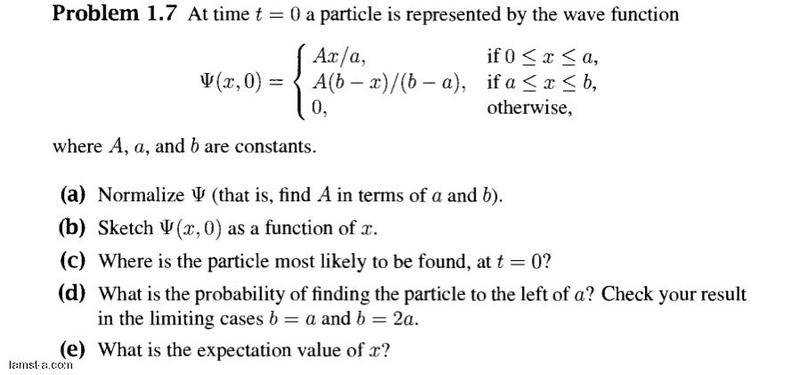# Quantum mechanics: how to sketch the wave function?

Fatimah od

## Homework Statement## Homework Equations

The solution which I found:

http://www.lamst-a.net/upfiles/mRA51397.png

## The Attempt at a Solution

I tried to solve part (a)

http://www.lamst-a.net/upfiles/wFU51621.jpg

Last edited by a moderator:

voko

I don't see how the solution you found corresponds to the problem.

Your solution is incorrect because you ignore the function in [0, a].

Fatimah od

so should i do the same integrate with the both intervales ?

voko
Compute the integral over the entire range [0, b]. Equate the result with 1 and solve it for A.

Fatimah od
http://im26.gulfup.com/2012-09-13/1347559112201.jpg [Broken]

how is it now !

Last edited by a moderator:
voko
I do not see any difference from the previous attempt. You are still not taking the entire [0, b] range.

Fatimah od
sorry I upload the wrong pic.

hear is the whole range.

http://im24.gulfup.com/2012-09-13/1347562820501.jpg [Broken]

I hope that I'm doing well.

Last edited by a moderator:
voko
This seems OK.

Staff Emeritus
Homework Helper

Fatimah od
fine,, thanx

so how should I sketch the function now ?!

Staff Emeritus
Homework Helper
Put to use what you learned in your algebra classes and plot the function.

Fatimah od
Iam trying ,,
is the time on the (x-axis)
and the position on the (y-axis) ?

should I write two tabels for the both cases individual !

voko
You are asked to sketch the function at t = 0 as a function of x.

Fatimah od
while i asked to sketch at t=0 , so i will use the first function ..

http://im18.gulfup.com/2012-09-13/1347568161121.jpg [Broken]

i Supposed a random numbers ,,

how is it ?!

Last edited by a moderator:
voko
You need to sketch this on the interval [0, b]. With a somewhere in between. Random numbers are useless.

Fatimah od
sorry
can you explain more please ,,
how could I draw without a points !

voko
On the x axis, choose some interval. Label its left end "0", its right end "b". Somewhere in that interval, choose another point, label it "a". What are the values of the function at those three points? Put them on them on the diagram. What else do you need to complete the sketch?

Fatimah od
how to chose the values of the position,which located on the y-axis ?

voko
I do not understand the question.

Fatimah od
I am trying to say that ,,
if the time will label on the x-axis with 3 points (0,a,b)
what is the functions which should label on the y-axis ?

voko
As I already said, there is no time in this problem. As to what labels you should use on the y axis, consider what values you are going to plot.

Fatimah od
i'm keep trying , but I do not know why I did n't catch the idea !

is this right ?

http://www.gulfup.com/show/X356ozhua4h0kks [Broken]

Last edited by a moderator:
voko
What are the values of the function at 0, a and b?

Fatimah od
ψ = Ax/a
ψ = A (b-x)/(b-a)

i know that it's not available to put an (a variable) as a point ,,
that it's why I want to suppose a value for "a" and "b" !

voko
Those are not values of the function. Those are the function itself.

Fatimah od
huh ,, I confused !
would you like to draw an example ,, please !

voko
What is the value when x = 0? When x = a? When x = b?

Fatimah od
i think it's correct now ,,

http://www.gulfup.com/show/X9fk6ltca3fwoco [Broken]

^
^
i hope ()

Last edited by a moderator:
voko
No, that's not correct. You have one function, not two.

You have INTEGRATED the function over [0, b]. But you are unable to compute values of the same function in three points in the same interval. How is this possible?

Fatimah od
but the function is divided in the two interval , so how can I compute one function
I'm forced to express the function as two parts, I thought !

voko
Your function has ONE value at any ONE point x. Not two, not three, not any other number.

Fatimah od
you mean A=√3/b ?

voko
At what point is that the value of the function?

Fatimah od
at "A" from the integrated

Fatimah od
all what I need to draw is the values which are on the y-axis
and that is to describe the wave function ψ

the problem now is " what is the function on (0,b)"?

I'm not sure if I can summate the two parts from (0,a) to (a,b) without any other operator such as "integral" ?

actually I think it's not correct two ,, it leads to a complicated formulas !
but that's what I could think about to get one function as what you said ,,, !